Related Articles

# Class 10 RD Sharma Solutions – Chapter 12 Some Applications of Trigonometry – Exercise 12.1 | Set 2

• Last Updated : 08 Sep, 2021

### Question 27. A T.V. tower stands vertically on a bank of a river of a river. From a point on the other bank directly opposite the tower, the angle of elevation of the top of the tower is 60°. From a point 20 m away this point on the same bank, the angle of elevation of the top of the tower is 30°. Find the height of the tower and the width of the river.

Solution: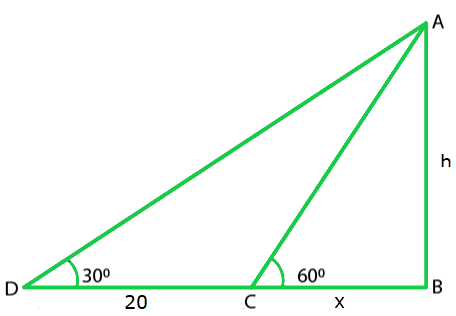Let us considered AB be the T.V tower of height ‘h’ m on the bank of river and

D be the point on the opposite side of the river.

So, the angle of elevation at the top of the tower is 30°

So, AB = h and BC = x

And, it is given that CD = 20 m

So,

In ΔACB

tan 60o = AB/BC

√3x = h

x = h/√3

Now, in ΔDBA,

tan 30o = AB/BD

1/√3 = h / (20 + x)

√3h = 20 + x

√3h – h/√3 = 20

2h/√3 = 20

h = 10√3 m

And,

x = h/√3

x = 10√3/ √3

x = 10

Hence, the height of the tower is 10√3 m and width of the river is 10 m.

### Question 28. From the top of a 7 m high building, the angle of elevation of the top of a cable is 60° and the angel of depression of its foot is 45°. Determine the height of the tower.

Solution: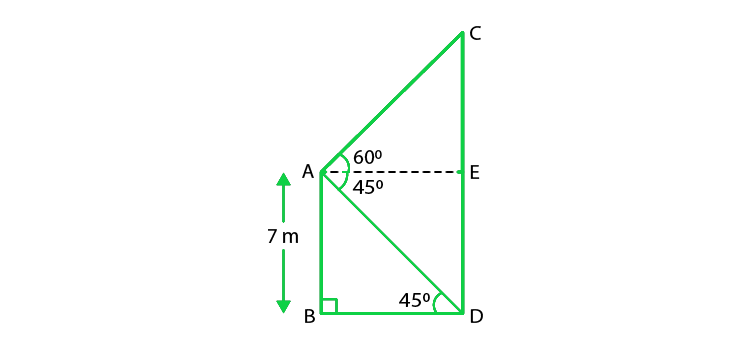Given that, the height of the building(AB) = 7 m

Let us considered the height of the cable tower is CD,

So, the angle of elevation of the top of the cable tower from the top of the building = 60°,

Angle of depression of the bottom of the building from the top of the building= 45°,

From the figure we conclude that,

ED = AB = 7 m

And,

CD = CE + ED

So, In ΔABD,

AB/ BD = tan 45°

AB = BD = 7

BD = 7

Now, in ΔACE,

AE = BD = 7

And, tan 60° = CE/AE

√3 = CE/ 7

CE = 7√3 m

So, CD = CE + ED = (7√3 + 7)= 7(√3 + 1) m

Hence, the height of the cable tower is 7(√3 + 1)m

### Question 29. As observed from the top of a 75 m tall lighthouse, the angles of depression of two ships are 30° and 45°. If one ship is exactly behind the other on the same side of the lighthouse, find the distance between the two ships.

Solution: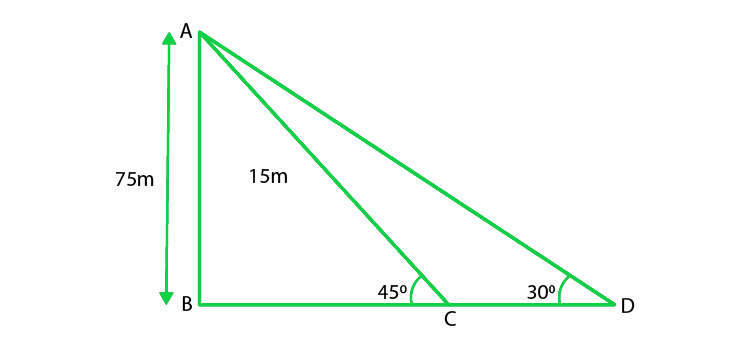Given that, the height of the lighthouse(AB) = 75m,

The angle of depression of ship 1, α = 30°,

The angle of depression of bottom of the tall building, β = 45°,

From the figure,

Let us considered distance between ships(CD) = x m,

So, In ΔABD,

tanα = AB/BD

tan 30o = 75/(x + BC)

x + BC = 75√3             ……(i)

So, In ΔABC,

tanβ = AB/BC

tan45o = 75/BC

BC = 75         ……(ii)

Now on substituting eq(ii) in eq(i), we get

x + 75 = 75√3

x = 75(√3 – 1)

Hence, the distance between the two ships is 75(√3 – 1) m

### Question 30. The angle of elevation of the top of the building from the foot of the tower is 30° and the angle of the top of the tower from the foot of the building is 60°. If the tower is 50 m high, find the height of the building.

Solution: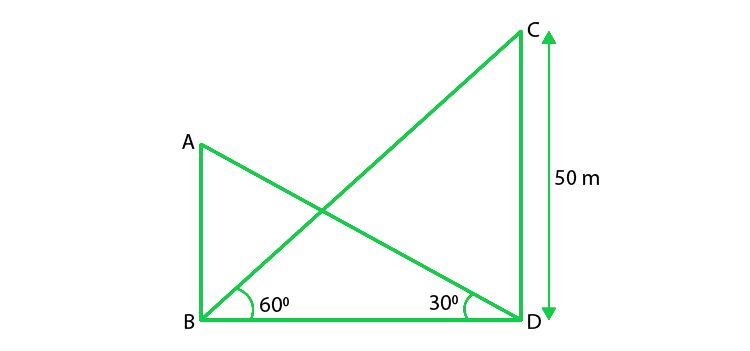Let’ us considered AB be the building and CD be the tower.

So, according to the question, given that

The angle of elevation of the top of the building from the foot of the tower = 30°,

And, the angle of elevation of the top of the tower from the foot of the building = 60°,

Height of the tower = CD = 50 m,

So, In ΔCDB,

CD/ BD = tan 60°

50/ BD = √3

BD = 50/√3 …. (i)

Next in ΔABD,

AB/ BD = tan 30°

AB/ BD = 1/√3

AB = BD/ √3

AB = 50/√3/ (√3)              (From eq(i))

AB = 50/3

Hence, the height of the building is 50/3 m.

### Question 31. From a point on a bridge across a river, the angle of depression of the banks on the opposite side of the river is 30° and 45° respectively. If the bridge is at the height of 30 m from the banks, find the width of the river.

Solution: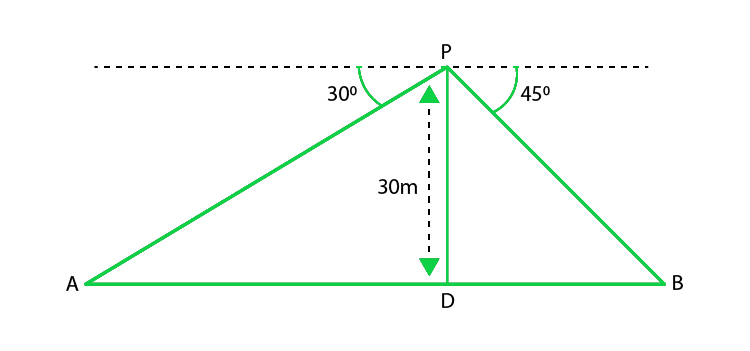According to the question, given that

The height of the bridge from the bank = 30 m

Let us considered A and B represent the points on the bank on opposite sides of the river.

So, AB is the width of the river.

Now, P is a point on the bridge which is 30 m high from the banks.

From the figure,

In ΔAPD,

Given that, ∠A = 30o

So, tan 30o = PD/ AD

Now, in ΔPBD,

∠B = 45o

So, tan 45o = PD/ BD

1 = PD/ BD

BD = PD

BD = 30 m

As we know that, AB = AD + DB = 30√3 + 30 = 30(√3 + 1)

Hence, the width of the river is 30(1 + √3) m

### Question 32. Two poles of equal heights are standing opposite each other on either side of the road which is 80 m wide. From a point between them on the road, the angles of elevation of the top of the poles are 60° and 30° respectively. Find the height of the poles and the distances of the point from the poles.

Solution: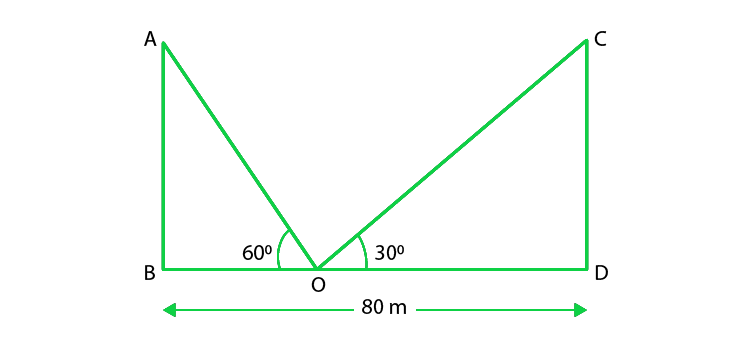According to the question, given that

The distance between the poles(BD) = 80 m

The angles of elevation to the top of the points is 60o and 30o

Let us considered AB and CD be the poles and O is the point on the road.

From the figure,

In ΔABO,

AB/ BO = tan 60°

AB/ BO = √3

BO = AB/ √3         …….(i)

So, in ΔCDO,

CD/ DO = tan 30o

CD/ (80 – BO) = 1/ √3

√3CD = 80 – BO

√3AB = 80 – (AB/√3)              (Since AB = CD and using eq(i))

3AB = 80√3 – AB

4AB = 80√3

AB = 20√3

So, BO = 20√3/(√3) = 20 m

And, DO = BD – BO = 80 – 20 = 60

Hence, the height of the poles is 20√3 m and

the distances of the points from the poles are 20m and 60 m.

### Question 33. A man sitting at a height of 20 m on a tall tree on a small island in the middle of a river observes two poles directly opposite each other on the two banks of the river and in line with the foot of the tree. If the angles of depression of the feet of the poles from a point at which the man is sitting on the tree on either side of the river are 60° and 30° respectively. Find the width of the river.

Solution: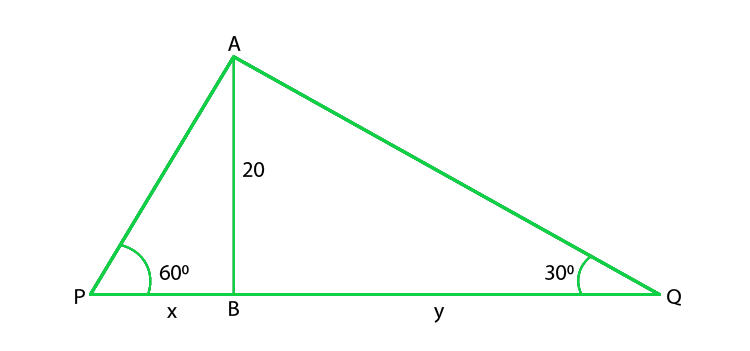From the given figure,

Let’s width of river = PQ = (x + y) m

Height of tree (AB) = 20 m

So, in ΔABP,

tan 60o = AB/ BP

√3 = 20/ x

x = 20/ √3 m

In ΔABQ,

tan 30o = AB/ BQ

1/ √3 = 20/ y

y = 20√3

So, (x + y) = 20/ √3 + 20√3

[20 + 20(3)]/ √3 = 80/√3

Hence, the width of the river is 80/√3 m.

### Question 34. A vertical tower stands on a horizontal plane and is surmounted by a flagstaff of height 7m. From a point on the plane, the angle of elevation of the bottom of the flagstaff is 30° and that of the top of the flagstaff is 45°. Find the height of the tower.

Solution: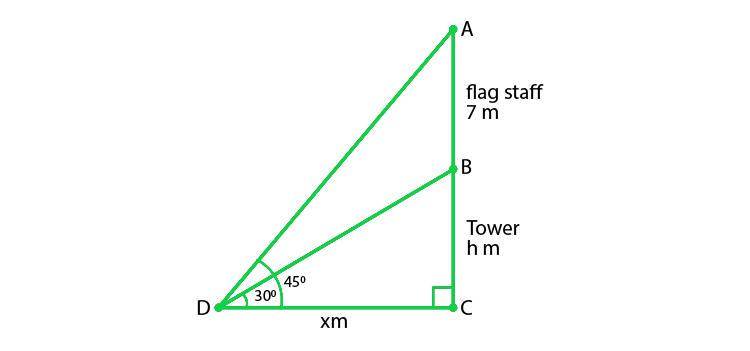According to the question,

The length of the flagstaff = 7 m

And the angles of elevation of the top and bottom of the flagstaff from point D are 45° and 30° respectively.

Let us considered the height of the tower (BC) = h m

And, DC = x m

So, in ΔBCD

tan 30° = BC/DC

1/ √3 = h/ x

x = h√3    ………(i)

And, in ΔACD

tan 45°  = AC/ DC

1 = (7 + h)/ x

x = 7 + h

h√3 = 7 + h                       (from eq(i))

h(√3 – 1) = 7

h = 7/(√3 – 1)

Now, on rationalizing the denominator we get

h = 7(√3 + 1)/ 2 = 7(1.732 + 1)/2 = 9.562

Hence, the height of the tower is 9.56 m

### Question 35. The length of the shadow of a tower standing on the level plane is found to 2x meters longer when the sun’s altitude is 30° than when it was 30°. Prove that the height of the tower is x(√3 + 1) meters.

Solution: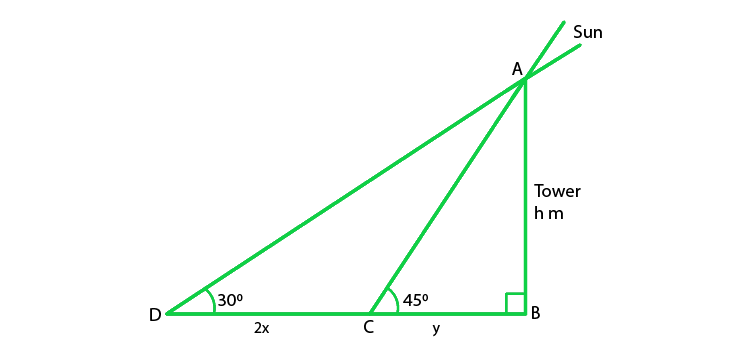According to the question,

CD = 2x, ∠D = 30°, and ∠C = 45°

Let us considered the height of the tower (AB) = h m,

And the distance BC = y m,

So, in ΔABC

tan 45° = AB/BC

1 = h/y

y = h

So, in ΔABD

tan 30° = AB/BD

1/√3 = h/ (2x + y)

2x + y = √3h

2x + h = √3h

2x = (√3 – 1)h

h = 2x/ (√3 – 1) x (√3 + 1)/ (√3 + 1)

h = 2x (√3 + 1)/(3-1) = 2x (√3 + 1)/2 = x (√3 + 1)

Hence, the height of the tower is x (√3 + 1) m

Hence Proved

### Question 36. A tree breaks due to a storm and the broken part bends so that the top of the tree touches the ground making an angle of 30° with the ground. The distance from the foot of the tree to the point where the top touches the ground is 10 meters. Find the height of the tree.

Solution: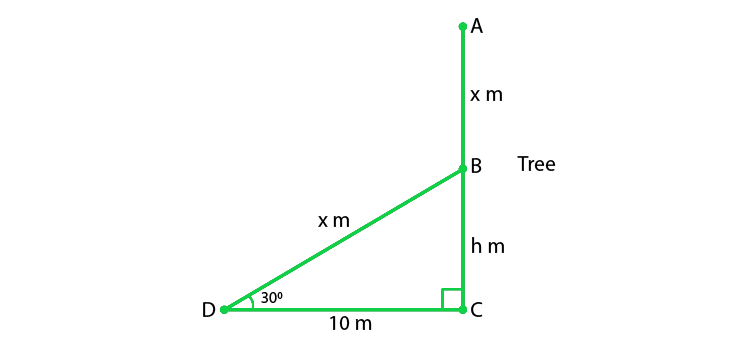Let us considered AC be the height of the tree which is (x + h) m

Given that, the broken portion of the tree is making an angle of 30° with the ground.

From the given figure,

In ΔBCD,

tan 30o = BC/ DC

1/√3 = h/ 10

h = 10/ √3

Now, in ΔBCD

cos 30o = DC/BD

√3/2 = 10/x

x = 20/√3 m

So,

x + h = 20/√3 + 10/√3 = 30/√3

10√3 = 10(1.732) = 17.32

Hence, the height of the tree is 17.32 m

### Question 37. A balloon is connected to a meteorological ground station by a cable of length 215 m inclined at 60° to the horizontal. Determine the height of the balloon from the ground. Assume that there is no slack in the cable.

Solution: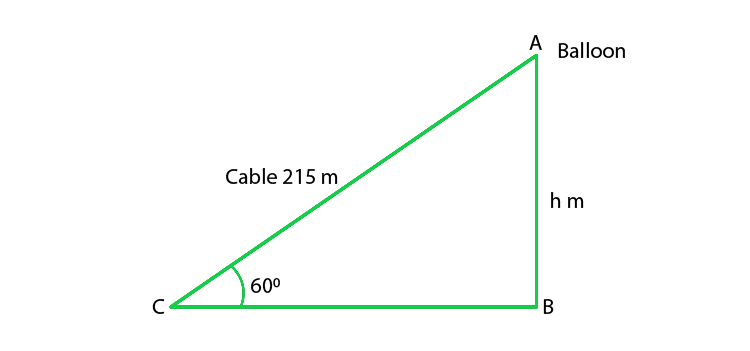Let us considered the height of the balloon from the ground = h m

Given that, the length of the cable = 215 m,

and the inclination of the cable is 60o.

So,

In ΔABC

sin 60o = AB/ AC

√3/2 = h/215

h = 215√3/2 = 185.9m

Hence, the height of the balloon from the ground is 186m (approx).

### Question 38. Two men on either side of the cliff 80 m high observe the angles of elevation of the top of the cliff to be 30° and 60° respectively. Find the distance between the two men.

Solution:

Let us considered CL be the cliff and A and B are two men on either side of the cliff

And they are making angles of elevation with C as 30° and 60°

It is given that, the height of cliff CL = 80 m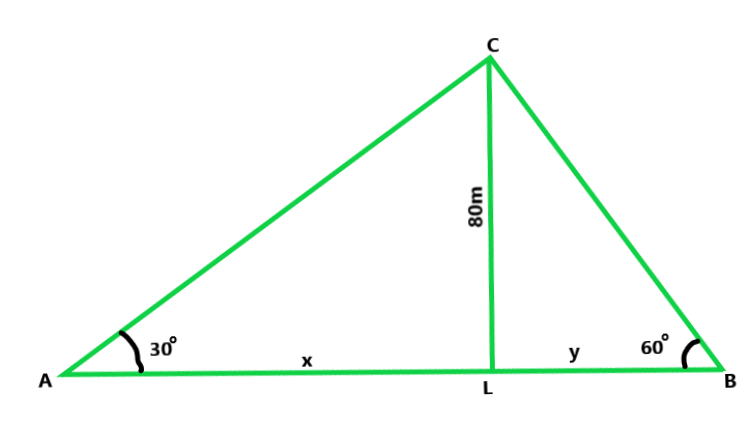Let us assume AL = x and BL = y

Now in ΔACL,

tan 30o = 80/x

x = 80√3

Similarly, in ΔBCL

tan 60o = CL/BL = √3 = 80/y

y = 80/√3

Hence, the distance between two men,

x + y = 80√3 + 80/√3

240+80 / √3 = 320/√3

On rationalize the value, we get,

320√3 / 3 = 184.746m

### Question 39. Find the angle of elevation of the sun (sun’s altitude) when the length of the shadow of a vertical pole is equal to its height.

Solution:

Let us considered the height of pole AB = h m,

Then the height of shadow = h m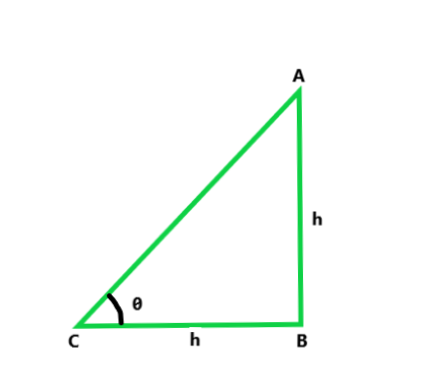Lets us considered the angle of elevation be θ,

So, In ΔABC,

tan θ = AB/BC = h/h = 1

tan θ = 1 = tan 45o

Hence, θ = 45o

### Question 40. An airplane is flying at a height of 210 m. Flying at this height at some instant the angles of depression of two points in a line in opposite directions on both the banks of the river are 45° and 60°. Find the width of the river. (Use √3  = 1.73)

Solution:

According to the question,

The height of the airplane = 210 m

Let us considered that, AB is the height of the airplane and

C and D are the points on the opposite banks of a river.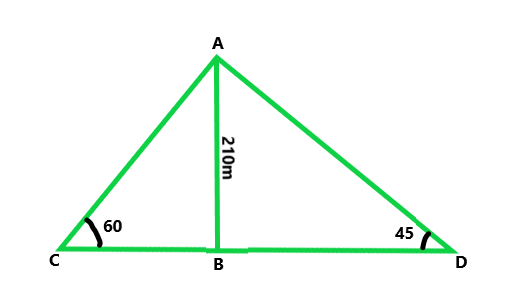So, the angle of elevation of A are 45° and 60°

Let us considered CB = x and BD = y

In ΔABD,

tan θ = P/B

tan 45o = AB/BD = 1 = AB/BD

210/BD = 1

BD = 210 m

Similarly, In ΔABC,

tan 60o = AB/CB = √3 = 210/CB

CB = 210/√3

On rationalize the value we get,

CB = 70√3 m = 121.10m

So, the width of river = CB + BD

= 121.10 + 210

= 331.10m

### Question 41. The angle of elevation of the top of a chimney from the top of a tower is 60° and the angle of depression of the foot of the chimney from the top of the tower is 30°. If the height of the tower is 40 m, find the height of the chimney. According to pollution control norms, the minimum height of a smoke-emitting chimney should be 100 m. State if the height of the above-mentioned chimney meets the pollution norms. What value is discussed in this question?

Solution:

Let us considered, CD be the tower and AB be the chimney

So, the angle of elevation of the top of the tower with the top of the chimney is 60° and

the foot of chimney with the top of the tower is 30°

So, it is given that CD = 40 m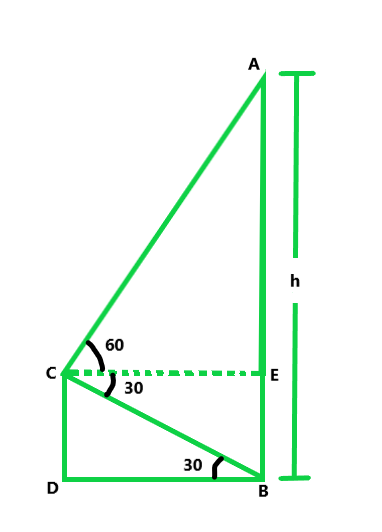From the given figure,

CE|| DB

So, CE = DB and EB = CD = 40

Now in △BCD

tan 30° = 40/DB = 1/√3

DB = 40√3             …….(i)

Now, in △ACE

tan 60° = AE/CE = √3 = (h – 40)/DB

DB = (h-40)/√3           …….(ii)

From eq(i) and (ii), we get

40√3 = (h – 40)/√3 = 120 + 40 = 160m

Hence, the height of the chimney is 160m.

### Question 42. Two ships are there in the sea on either side of a lighthouse in such a way that the ships and the lighthouse are in the same straight line. The angle of depression of the two ships are observed from the top of the lighthouse are 60° and 45° respectively. If the height of the lighthouse is 200 m, find the distance between the two ships. (Use √3 = 1.73)

Solution:

Let us considered that, AB be the lighthouse and

C and D are two ships which make an angle of depression

with the top A of the lighthouse of 60° and 45°

So, ∠C = 45° and ∠D = 60°

It is given that, AB = 20 m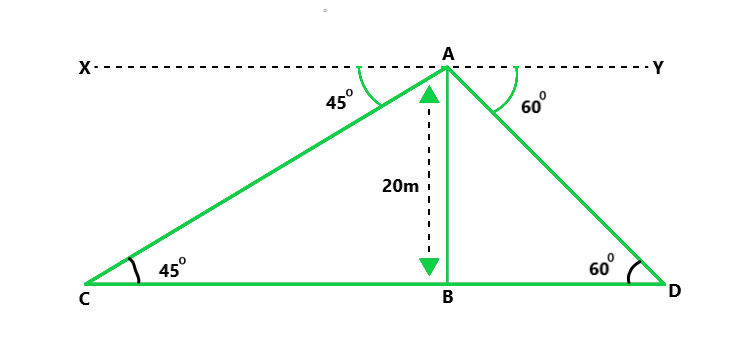Now in △ACB,

tan 45° = 20/CB

CB = 20m

Similarly, in △ABD,

tan 60° = AB/BD

√3 = 20/BD

BD = 20/√3 = 20√3/3 m

Now, the Distance between two ships

CD = CB + BD

20 + 20/3 √3 = 20(1 + √3)/3 m

= 20 x 0.19 = 18.20m = 18.2m

### Question 43. The horizontal distance between the two poles is 15 m. The angle of depression of the top of the first pole as seen from the top of the second pole is 30°. If the height of the second pole is 24 m, find the height of the first pole. (√3 = 1.732)

Solution:

Let us considered AB and CD are two poles and

the distance between them(BD) = 15 m,

The height of pole(AB) = 24 m

Angle of elevation from the top of pole CD, to pole AB = 30°

Now, from point C, draw CE || DB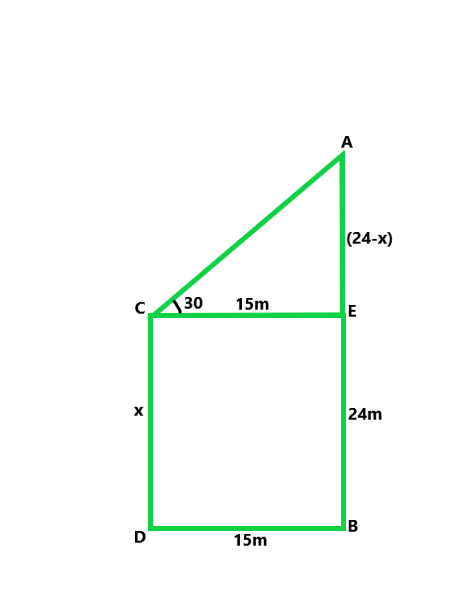Let us considered CD = x m,

CE = DB = 15m and AE = AB – EB

AE = AB – CD = (24 – x)m

Now in △ACE,

tan 30° = (24 – x)/15

1/√3 = (24 – x)/15

15 = √3 (24 – x)

x = 24 – 15/√3

Now, after rationalizing we get,

x = 15.34m

### Question 44. The angles of depression of two ships from the top of a lighthouse and on the same side are 45° and 30° respectively. If the ships are 200 m apart, find the height of the lighthouse.

Solution:

Let us considered PQ be the lighthouse and

A and B are two ships on the same side of the lighthouse

The angle of depression from the top of the lighthouse of the two ships are 30° and 45°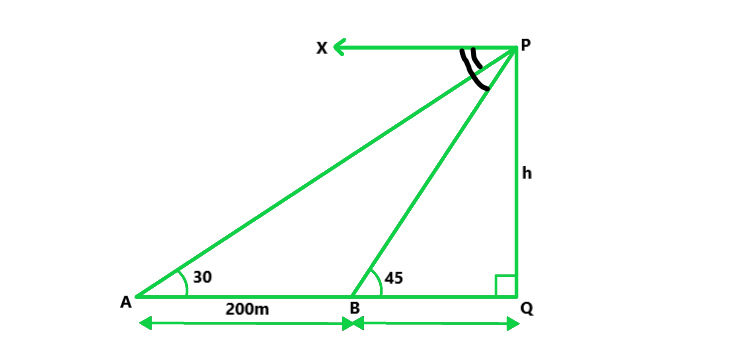So, ∠A = 30 and ∠b = 45 and AB = 200m

Now, let us considered the height of lighthouse = h,

and BQ = x

Now in △PBQ

tan 45° = h/x

h = x

Similarly, in △PAQ,

tan 30° = h/(200 + x)

1/√3 = h/(200 + h)

h(√3 – 1) = 200

h = 200/0.732 m = 273.2m

Hence, the height of the house is 273.2m.

### Question 45. The angles of elevation of the top of a tower from two points at a distance of 4 m and 9 m from the base of the tower and in the same straight line with it are complementary. Prove that the height of the tower is 6 m.

Solution:

Let us considered TR be the tower.

A and B are two points which make the angled elevation with top of the tower as θ and 90° – θ (∵ angles are complementary)

Also, the height of tower TR = h and AR = 9 m, BR = 4m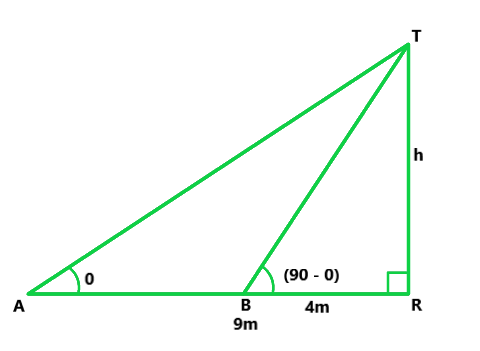Now in △TAR

tan θ = TR/AR = h/9      ……(i)

Similarly, in right △TBR,

tan(90 – θ) = h/4 = cot θ = h/4    ……(ii)

Now, on multiplying eq(i) and (ii), we get

tan θ x cot θ = h/4 x h/9

1 = h2/36

h2 = 36

h = 6m

Hence, height of tower is 6m

### Question 46. From the top of a 50 m high tower, the angles of depression of the top and bottom of a pole are observed to be 45° and 60° respectively. Find the height of the pole.

Solution:

Let us considered AB be the tower and CD is the pole.

So, the angles of depression from the top A to the top and bottom of the pole are 45° and 60°

AB = 50 m,

Let us considered CD = h and BD = EC = x

∵ CE || DB

∴ EB = CD = h

and AE = 50 – h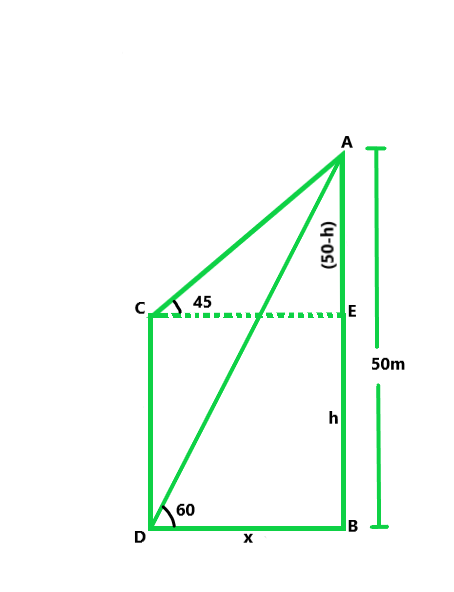tan 60° = 50/x

√3 = 50/x

x = 50/√3            ……(i)

Similarly, in △ACE,

tan 45° = AE/CE = 1 = (50 – h)/x

x = 50 – h

x + h = 50

h = 50 – x

50 – (50/√3) = 21.13m             (using eq(i))

Hence, the height of pole is 21.13m

### Question 47. The horizontal distance between two trees of different heights is 60 m. The angle of depression of the top of the first tree, when seen from the top of the second tree, is 45°. If the height of the second tree is 80 m, find the height of the first tree.

Solution:

Let us considered AB and CD be the two trees

So, given that AB = 80 m, angle of depression from A to C is 45°.

Now, draw CE || DB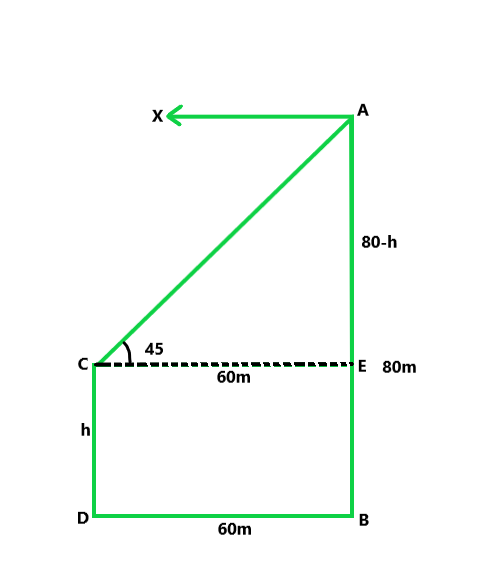∠ACE = ∠XAC = 45°

Let us assume CD = h

CE = BD = 60 m

EB = CD = h and AE = 80 – h

Now in right △ACE

tan 45° = (80 – h)/60

80 – h = 60

h = 20m

Hence the height of the second tree is 20m.

### Question 48. A flag-staff stands on the top of a 5 m high tower. From a point on the ground, the angle of elevation of the top of the flag-staff is 60° and from the same point, the angle of elevation of the top of the tower is 45°. Find the height of the flag­staff.

Solution:

Let us considered FT is the flag-staff situated on the top of the tower TR.

Now, A is any point on the same plane that makes elevation angles with

top of the flag-staff and top of the tower are 60° and 45°.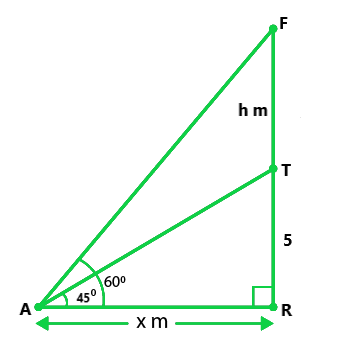Given that the length of tower TR = 5m

Let us assume that the height of flag-staff FT = h and AR = x, then

In △TAR,

tan 45° = 5/x

x = 5           …..(i)

Similarly in △FAR,

tan 60° = FR/AR = √3 = (h + 5)/x

x = (h + 5)/√3           ……(ii)

Now, from eq(i) and (ii), we get

5 = (h + 5)/√3

5√3 = h + 5

h = 5(√3 – 1) = 3.65m

Hence the height of the flag-staff is 3.65m.

### Question 49. The angle of elevation of the top of a vertical tower PQ from a point X on the ground is 60°. At a point Y, 40 m vertically above X, the angle of elevation of the top is 45°. Calculate the height of the tower.

Solution:

Let us considered that TR is the tower .

Now, from a point X, the angle of elevation of T is 60° and 40 m

above X, from the point Y, the angle of elevation is 45°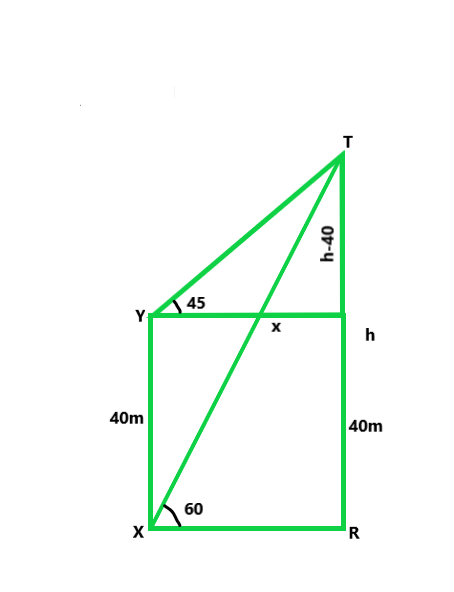From Y, draw YZ || XR

Let us considered, TR = h and XR = YZ = x

ZR = YX = 40m and TZ = (h – 40) m

In △TXR,

tan 60° = h/x

√3 = h/x

x = h/√3              ……(i)

Similarly in △TYZ,

tan 45° = TZ/YZ

1 = (h – 40)/x

x = h – 40              ……(ii)

From eq(i) and (ii), we get

h/√3 = h – 40

h(√3 – 1) = 40√3

h = 40√3/(√3 – 1)

On rationalize above term and we get,

h = 94.64m

Hence, the height of the tower is 94.64m

### Question 50. As observed from the top of a 150 m tall lighthouse, the angles of depression of two ships approaching it are 30° and 45°. If one ship is directly behind the other, find the distance between the two ships.

Solution:

Let us considered, LH be the lighthouse,

So, A and B are the two ships making angles of elevation

with the top of the lighthouse as 30° and 45°.

LH = 150 m.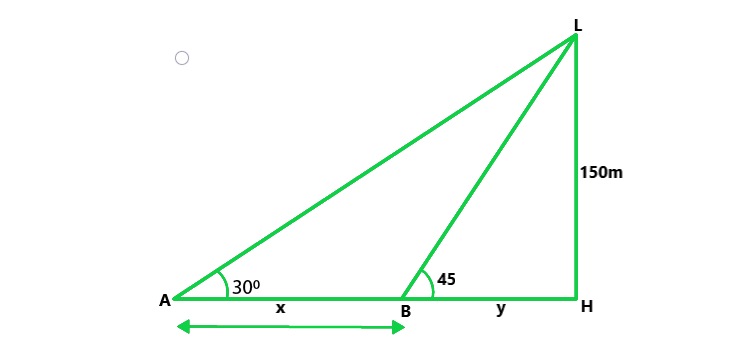Let us considered AB = x and BH = y

Now in △LAH,

tan 30° = 150/(x + y)

1/√3 = 150/(x + y)            ……..(i)

Similarly in △LBH,

tan 45° = LH/BH

1 = 150/y

y = 150  ……(ii)

From eq(i), we get

x + 150 = 150√3

x = 150√3 – 150

= 109.5 m

Hence the Distance between two ships is 109.5 m.

### Question 51. The angles of elevation of the top of the rock from the top and foot of a 100 m high tower are respectively 30° and 45°. Find the height of the rock.

Solution:

Let us considered RS is the rock and TP is the tower.

So, the angles of elevation of the top of the rock with

the top and foot of the tower are 30° and 45°.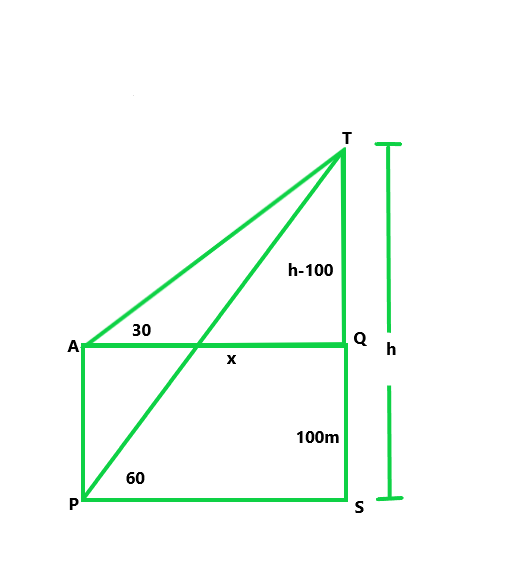Given that, the height of TP = 100 m

Let the height of rock RS = h

From T, draw TQ || PS

Then QS = TP = 100 m

and RQ = h – 100

Let us assume, PS = TQ = x

Now in right ΔRPS,

tan 45° = h/x

x = h  …..(i)

Similarly in △RTQ,

tan 30° = RQ/TQ = 1/√3 = (h – 100)/x

x = √3(h – 100)     ….(ii)

From eq(i) and (ii), we get

h = √3(h – 100)

h(√3 – 1) = 100√3

On rationalize above value we get,

h = 50√3(√3 – 1) = 236.5 m

Hence the height of rock is 236.5 m.

### Question 52. A straight highway leads to the foot of a tower of height 50 m. From the top of the tower, the angles of depression of two cars standing on the highway are 30° and 60° respectively. What is the distance between the two cars and how far is each car from the tower?

Solution:

Let us considered, TR be the tower

And A and B are two cars on the road making angles of elevation

with T the top of the tower as 30° and 60°

Height of the tower TR = 50 m

Let us considered AR = x and BR = y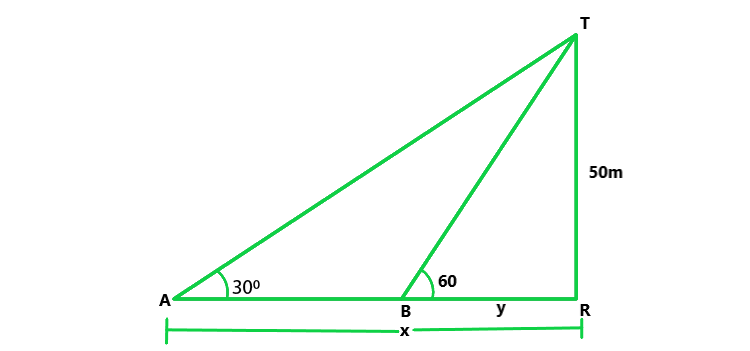Now in ΔTAR,

tan 30° = TR/AR = 50/x

1/√3 = 50/x

x = 50√3         …….(i)

Similarly in △TRB,

tan 60° = TR/BR = 50/y

√3  =50/y

y = 50/√3       …..(ii)

(i) Now we find the distance between the two cars

AB = AR – BR = x – y

= (50√3 – 50/√3) = 50(√3 – 1/√3)

= 50((3 – 2) / √3) x (50 x 2)/√3

On rationalize above term and we get,

AB = 57.7 m

(ii) Now we find the how far is each car from the tower

Distance of A car = x = 50√3 = 86.65 m

Distance of B car = y = 50/√3 = 50√3/3 = 28.83 m

Attention reader! All those who say programming isn’t for kids, just haven’t met the right mentors yet. Join the  Demo Class for First Step to Coding Coursespecifically designed for students of class 8 to 12.

The students will get to learn more about the world of programming in these free classes which will definitely help them in making a wise career choice in the future.

My Personal Notes arrow_drop_up